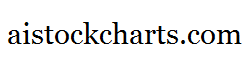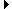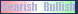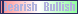Members AreaSign Up | Log In
 Free Level 2 QuotesStock AnalysisCorrelation Trading PlatformCorrelationsMembership Benefits

IMI
 Lookup a different indicator.

 Indicator Usage Description Example imi imi[x,n] OPERATOR (INDICATOR or NUMBER) , where x is a positive integer >= 2 and n is optional and represents the number of days ago the result is computed (n=0 is the default value and represents the current end of day, n=1 is the previous end of day, etc.) Returns the x-day intraday momentum index from n days ago. (imi < 30)

Introduction:

The IMI (intraday momentum index) is a ratio of the total magnitude of the up day candle bodies to the total magnitude of the down day candle bodies over the past x-days. An up day candle body is white and is the close minus the open for a particular day. A down day candle body is black and is the open minus the close for a particular day. So if you see a lot of consecutive long white candle bodies and only a few short black body candle bodies on a stock chart then the IMI will be high (perhaps near 100%). The 'imi' indicator is used in indicator formula construction to narrow the stock pre-screener results to include only those stocks that have an imi restricted to the parameters set for the imi indicator.

Example:

The following chart of Intel (INTC) highlights days where the 14-day intraday momentum index is less than 30%.

 Chart of Intel (INTC) highlighting days where the 14-day intraday momentum index is less than 30%.

INDICATORS IN OUR DATABASE THAT INCLUDE THE IMI INDICATOR...

Click here to view all indicators that include the indicator 'imi' (sorted by AISCORE)

 (imi < 30)AISCORE: 5462Indicator ID: 060310143034(high < high) and (high < high) and (low < low) and (low < low) and (imi < 30)AISCORE: 6689Indicator ID: 060322223706(high < high) and (high < high) and (low < low) and (low < low) and (faststo[25,3,d] < 20) and (black) and (imi < 50)AISCORE: 7087Indicator ID: 060322225309(lgbody) and (black) and (imi < 30)AISCORE: 6005Indicator ID: 060506112725(p3range > 10) and (p4change < -2) and (imi < 40)AISCORE: -3637Indicator ID: 060811180000 More ->